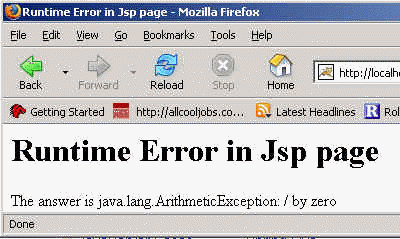Tutorials

Runtime Errors

Errors are arised when there is any logic problem with the logic of the program. In this program we are trying to divide the number by zero which results in runtime error.

Errors are arised when there is any logic problem with the logic of the program. In this program we are trying to divide the number by zero which results in runtime error.

Runtime Errors

Errors are arised when there is any logic problem with the logic of the program.

In this program we are trying to divide the number by zero which results in runtime error.

The code of the program is given below:

 Runtime Error in Jsp page

Runtime Error in Jsp page

<% try{ int a = 10; int b = 10; b = a / 0; } catch(Exception e){ out.println("The answer is " + e); } %>

Output of the Program: